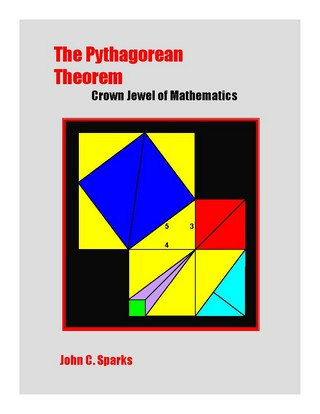### Book Toankho

Sách hay - Sách đẹp

# The Pythagorean Theorem (Crown Jewel of Mathematics)The Pythagorean Theorem, Crown Jewel of Mathematics is a general introduction to the Pythagorean Theorem and its many applications throughout mathematics. The book includes a historical development of the Pythagorean Theorem via a series of proofs that increase in sophistication as centuries progress. Also within the book are chapters addressing mathematical spinoffs including trigonometry, puzzles, and pastimes.